# Value

Determine the value of the following exspressions:

a) (23-25)·(4-5)
b) (97-123):(18+8)

Result

a =  2
b =  -1

#### Solution:Leave us a comment of example and its solution (i.e. if it is still somewhat unclear...):Be the first to comment!## Next similar examples:

1. What isWhat is the value of the smaller of a pair of numbers for which their sum is 78 and their division quotients are 0.3?
2. Women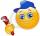In an company of 1050 employees are 2/3 women. How many women work in the company?
3. Balls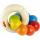The boys changed stamps, beads and balls. For 9 balls is 3 stamps, 2 balls is 44 stamps. How many beads is for 1 ball?
4. Products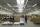15 products are 48 € more expensive than 12 products. How many euros will it cost five same products?
5. Expression plus minusEvaluate expression: (-1)2 . 12 – 6 : 3 + (-3) . (-2) + 22 – (-3) . 2
6. NumberCalculate the integer number which, divided by 34 gives 10 and the rest 25.
7. Wallpaper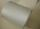Salesman account for 2.3 meters of wallpaper 149.50 Kc. How much cost 1 meter of wallpaper?
8. ProductResult of the product of the numbers 1, 2, 3, 1, 2, 0 is:
9. Icecreams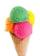Karthik bought 30 icecreams for his birthday .2/5 of them are chocolate icecreams. Find the number of chocolate icecreams he bought?
10. Computer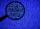A line of print on a computer contains 64 characters (letters, spacers or other chars). Find how many characters there are in 7 lines.
11. Decimal expansion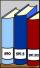Calculate: 2 . 1 + 0 . 10 + 7 . 10000 + 4 . 1000 + 6 . 100 + 0 . 100000 =
12. DoctorsIn the city operates 171 doctors. The city has 128934 citizens. How many citizens are per one doctor?
13. On the farm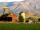The farm took 221 tons of potatoes from 17 hectares. How many tons of potatoes taken from one hectare?
14. The resultHow many times I decrease the number 1632 to get the result 24?
15. Dividing by five and tenNumber 5040 divide by the number 5 and by number 10: a = 5040: 5 b = 5040: 10
16. Math classificationIn 3A class are 27 students. One-third got a B in math and the rest got A. How many students received a B in math?
17. Trio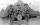56 children lined up in groups of three. How many children did not create a trio?